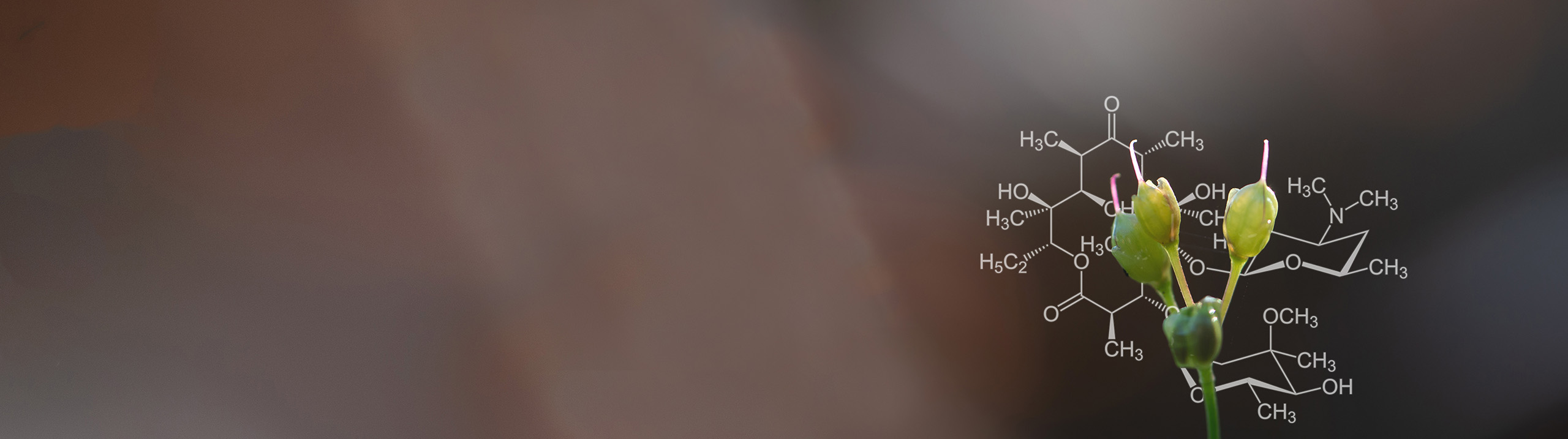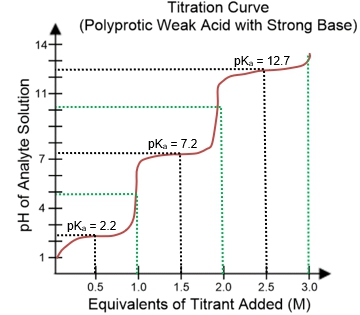Start typing, then use the up and down aroows to select an option from the list.# Biochemistry

Learn the toughest concepts covered in Biochemistry with step-by-step video tutorials and practice problems by world-class tutors

2. Water
1

### concept

Titrations7m
Play a video:
1
2
Problem

Which of the following titration curves expresses the titration of a weak acid with a strong base?

3

### concept

Titration of Monoprotic Weak Acids4m
Play a video:
0
4
Problem

You have an analyte solution of 50 mL of 0.2 M acetic acid (pKa = 4.8). What volume of 0.05 M NaOH titrant needs to be added to get the final pH = pKa?

5

### concept

Titration of Polyprotic Weak Acids6m
Play a video:
0
6
Problem

Use the titration curve below.What is the predominate species in the solution of phosphoric acid at pH = 5?

7
Problem

Titration confirms an acetic acid solution to be 0.1 M. Calculate the pH. (acetic acid K a = 1.76 x 10-5 M).